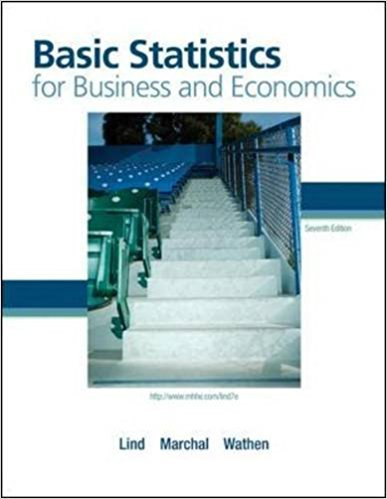×
×

# Solutions for Chapter 4: Basic Statistics for Business and Economics 7th Edition## Full solutions for Basic Statistics for Business and Economics | 7th Edition

ISBN: 9780077384470Solutions for Chapter 4

Solutions for Chapter 4
4 5 0 322 Reviews
30
2
##### ISBN: 9780077384470

Since 36 problems in chapter 4 have been answered, more than 81486 students have viewed full step-by-step solutions from this chapter. This textbook survival guide was created for the textbook: Basic Statistics for Business and Economics , edition: 7. This expansive textbook survival guide covers the following chapters and their solutions. Basic Statistics for Business and Economics was written by and is associated to the ISBN: 9780077384470. Chapter 4 includes 36 full step-by-step solutions.

Key Business Terms and definitions covered in this textbook

the tendency for the mix of unobserved attributes to become undesirable from the standpoint of an uninformed party

• average variable cost

variable cost divided by the quantity of output

• consumer price index (CPI)

a measure of the overall cost of the goods and services bought by a typical consumer

• cost

the value of everything a seller must give up to produce a good

the fall in total surplus that results from a market distortion, such as a tax

• demand curve

a graph of the relationship between the price of a good and the quantity demanded

• economic profit

total revenue minus total cost, including both explicit and implicit costs

• efficiency wages

above-equilibrium wages paid by firms to increase worker productivity

• efficient scale

the quantity of output that minimizes average total cost

• indifference curve

a curve that shows consumption bundles that give the consumer the same level of satisfaction

• marginal tax rate

the amount that taxes increase from an additional dollar of income

• market economy

an economy that allocates resources through the decentralized decisions of many firms and households as they interact in markets for goods and services

• model of aggregate demand and aggregate supply

the model that most economists use to explain shortrun fluctuations in economic activity around its long-run trend

• monetary policy

the setting of the money supply by policymakers in the central bank

• Nash equilibrium

a situation in which economic actors interacting with one another each choose their best strategy given the strategies that all the other actors have chosen

• natural monopoly

a monopoly that arises because a single firm can supply a good or service to an entire market at a smaller cost than could two or more firms

• political economy

the study of government using the analytic methods of economics

• quantity equation

the equation M × V = P × Y, which relates the quantity of money, the velocity of money, and the dollar value of the economy’s output of goods and services

• shortage

a situation in which quantity demanded is greater than quantity supplied

• store of value

an item that people can use to transfer purchasing power from the present to the future

×